# DC Motor Control Using MATLAB and Arduino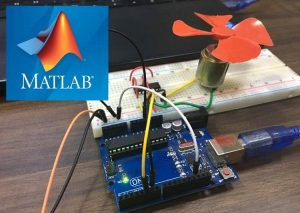In this tutorial, we will show you how to control DC motor using MATLB and Arduino. If you are new with MATLAB then it is recommend to get started with simple LED blink program with MATLAB.

### Creating MATLAB Graphical User Interface for controlling DC Motor

After finishing setup with Arduino for MATLAB, we have to build GUI (Graphical User Interface) to control DC motor. To launch the GUI, type the below command in the command window

guide

A popup window will open, then select new blank GUI as shown in below image,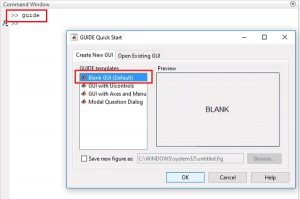Now choose three pushbuttons for Clockwise rotation, Anti-clockwise rotation and STOP, as shown below,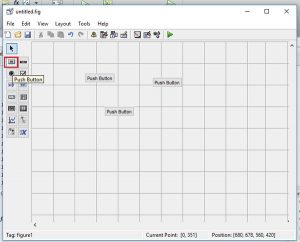To resize or to change the shape of the button, just click on it and you will be able to drag the corners of the button. By double-clicking on pushbutton you can change the color, string and tag of that particular button. We have customized three buttons as shown in below picture.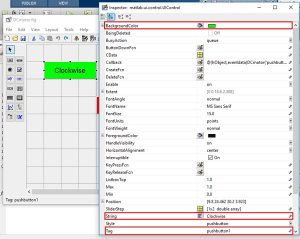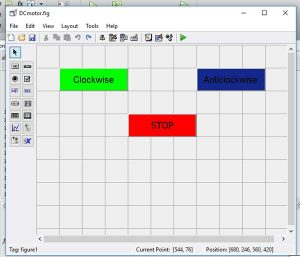You can customize the buttons as per your choice. Now when you save this, a code is generated in the Editor windowof MATLAB. To code your Arduino for performing any task related to your project, you always have to edit this generated code. So below we have edited the MATLAB code.

### MATLAB Code for controlling DC Motor with Arduino

Complete MATLAB code, after editing it for DC motor control, is given at the end of this project. Further we are including the GUI file (.fig) and code file(.m) here for download, using which you can customize the buttons as per your requirement. Below are some tweaks we did for rotating the DC Motor clockwise, anticlockwise and stop using three push buttons.Copy and paste the below code on line no. 74 to make sure that the Arduino is talking with MATLAB every time you run the m-file.

clear all;
global a;
a = arduino();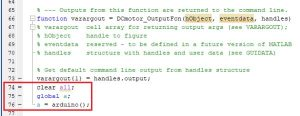When you scroll down, you will see that there are three functions for every Button in the GUI. Now write the code in every function according to task you want to perform on click.

In Clockwise button’s function, copy and paste the below code just before the ending braces of the function to rotate the motor in clockwise direction. Here we are giving HIGH at pin 6 and LOW at pin 5 to rotate the motor in clockwise direction.

global a;
writeDigitalPin(a, ‘D5’, 0);
writeDigitalPin(a, ‘D6’, 1);
pause(0.5);Now in Anti-clockwise button’s function, paste the below code at the end of the function to rotate the motor in anti-clockwise direction. Here we are giving HIGH at pin 5 and LOW at pin 6 to rotate the motor in Anti-clockwise direction.

global a;
writeDigitalPin(a, ‘D5’, 1);
writeDigitalPin(a, ‘D6’, 0);
pause(0.5);Finally in STOP button’s function, paste the below code at the end, to stop the rotation of motor. Here we are giving LOW at both pin 5 and 6 to stop the motor.

global a;
writeDigitalPin(a, ‘D5’, 0);
writeDigitalPin(a, ‘D6’, 0);
pause(0.5);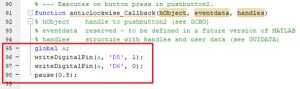### Material Required

• MATLAB installed Laptop (Preference: R2016a or above versions)
• Arduino UNO
• DC Motor
• L293D- motor driver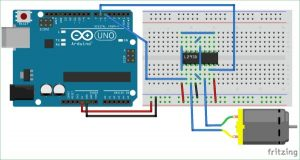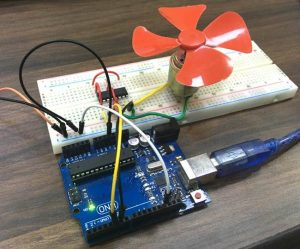### Controlling DC Motor with MATLAB

After setup the hardware according to circuit diagram, just click on the run button to run the edited code in .m file#### This Post / Project can also be found using search terms:

• arduino dc control matlab